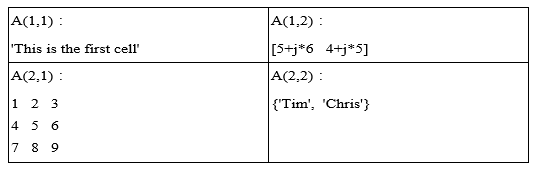12-1 嚙踝蕭嚙踝蕭嚙踝蕭嚙踝蕭嚙踝蕭嚙踝蕭嚙踝蕭嚙踝蕭嚙踝蕭嚙踝蕭嚙?

Hint
Cell Arrays 照英文字面翻譯應該是「盒子陣列」或是「胞陣列」，但是其意義並不明顯。因此筆者在此將其翻譯為「異質陣列」，取其陣列各個構成元素可以包含「不同性質的資料型態」的意義。

Example 1: 12-異質陣列/cell01.mA(1,1) = {'This is the first cell.'}; A(1,2) = {[5+j*6 , 4+j*5]}; A(2,1) = {[1 2 3; 4 5 6; 7 8 9]}; A(2,2) = {{'Tim'; 'Chris'}} A = 'This is the first cell.' [1x2 double] [3x3 double] {2x1 cell }Example 2: 12-異質陣列/cell02.mA{1,1} = 'this is the first cell.'; A{1,2} = [5+j*6, 4+j*5]; A{2,1} = [1 2 3; 4 5 6; 7 8 9]; A{2,2} = {'Tim'; 'Chris'} A = 'this is the first cell.' [1x2 double] [3x3 double] {2x1 cell }

• A(2, 3) = 5 代表一般矩陣 A的第二列、第三行元素為 5。
• A{2, 3} = [12, 34] 代表異值陣列 A 的第二列、第三行元素為 [12, 34]。

Example 3: 12-異質陣列/cellConcat01.mA = {'James Bond', [1 2;3 4;5 6]; pi, magic(5)} B = {rand(3), randperm(4); eps, 'NTU'} C = [A B] % 將異質陣列 A 及 B 左右並排 A = 'James Bond' [3x2 double] [ 3.1416] [5x5 double] B = [3x3 double] [1x4 double] [2.2204e-16] 'NTU' C = 'James Bond' [3x2 double] [3x3 double] [1x4 double] [ 3.1416] [5x5 double] [2.2204e-16] 'NTU'

MATLAB程式設計：入門篇